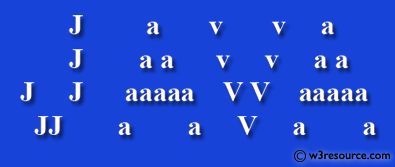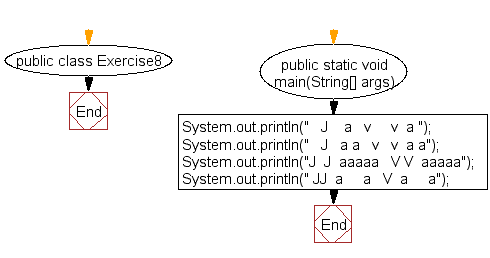﻿ Java exercises: Display a specified pattern - w3resource# Java Exercises: Display a specified pattern

## Java Basic: Exercise-8 with Solution

Write a Java program to display the following pattern.

Sample Pattern :

```   J    a   v     v  a
J   a a   v   v  a a
J  J  aaaaa   V V  aaaaa
JJ  a     a   V  a     a
```

Pictorial Presentation:Sample Solution:

Java Code:

``````public class Exercise8 {

public static void main(String[] args) {

System.out.println("   J    a   v     v  a ");
System.out.println("   J   a a   v   v  a a");
System.out.println("J  J  aaaaa   V V  aaaaa");
System.out.println(" JJ  a     a   V  a     a");
}
}
```
```

Sample Output:

```  J    a   v     v  a
J   a a   v   v  a a
J  J  aaaaa   V V  aaaaa
JJ  a     a   V  a     a
```

Flowchart:Java Code Editor:

What is the difficulty level of this exercise?

Test your Programming skills with w3resource's quiz.

﻿

## Java: Tips of the Day

countOccurrences

Counts the occurrences of a value in an array.

Use Arrays.stream().filter().count() to count total number of values that equals the specified value.

```public static long countOccurrences(int[] numbers, int value) {
return Arrays.stream(numbers)
.filter(number -> number == value)
.count();
}
```

Ref: https://bit.ly/3kCAgLb